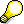Formulas for Calculating Setup, Processing and TeardownThe system calculates the duration of the operation segments that make up the execution time (setup, processing and teardown) using formulas. You can enter a separate formula for each of these three operation segments in the work center. You can use the following parameters in the formulas:

• standard values depending on the standard value key
• formula constants from the work center
• general operation values, such as
• lot size
• base quantity
• number of splits (partial lots)
• values from user-defined fields in the operationIf you have not maintained a formula or standard values for a formula to calculate duration times for one of the three operation segments (setup, processing or teardown), the system calculates for the corresponding operation segment using a duration of zero.

The formulas used to calculate the duration are not the same as those used to calculate capacity requirements. They are maintained separately in the work center. The system can only calculate the duration of the individual operation segments if you have maintained the formulas on the Scheduling screen in the work center.To calculate the setup, processing and teardown times of an operation, the system always uses the smallest unit for the standard values. If you want the times to be calculated using a larger unit, you must change the smallest time unit of the standard values.